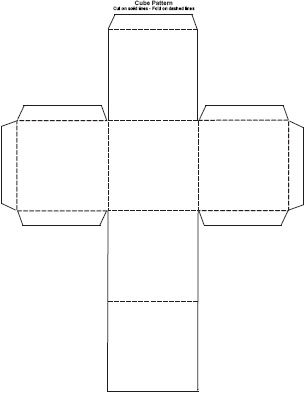## Friday, December 11, 2009

### The Cube A Differentiated Instruction LessonIn my Introduction to Algebra Class we did a lesson covering the area of a triangle, rectangle, and a parallelogram. We used a differentiated lesson idea of making a cube. The students wrote questions on 5 sides of the cube and then their answers on the 6th side of the cube. On the five sides students had a choice of what types of question s to put on it. They could choose easy, medium or difficult questions. The students would then use scissors to cut out and tape together their cube. Once the students were done with making the cube, they found another student to roll the cube with. They would then they would do the problem and then check with the answer side of the cube. It turned out to be a great activity of choosing problems and checking their answers. I have attached a link to the the activity that I did.

The activity
A PDF Cube